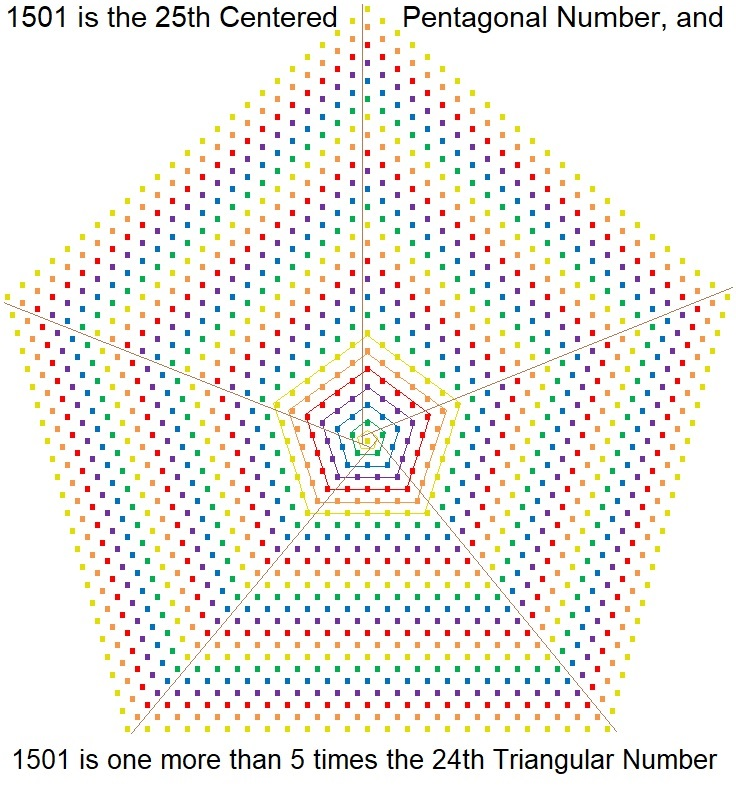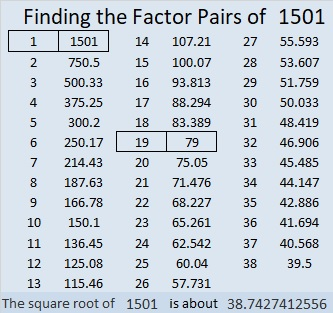# 1501 is a Centered Pentagonal Number

### 1501 Tiny Dot Puzzle

A long time ago before calculators or computers existed, someone determined that 1501 tiny dots could be formed into a pentagon. That was a remarkable puzzle to complete! What kind of pentagon will 1501 tiny dots make?

Since 5(24)(25)/2 + 1 = 1501, it is the 25th centered pentagonal number.  Notice in the graphic below that those 1501 tiny dots can also be divided into 5 equally-sized triangles with just the center dot leftover.### Factors of 1501:

• 1501 is a composite number.
• Prime factorization: 1501 = 19 × 79.
• 1501 has no exponents greater than 1 in its prime factorization, so √1501 cannot be simplified.
• The exponents in the prime factorization are 1, and 1. Adding one to each exponent and multiplying we get (1 + 1)(1 + 1) = 2 × 2 = 4. Therefore 1501 has exactly 4 factors.
• The factors of 1501 are outlined with their factor pair partners in the graphic below.### Another fact about the number 1501:

1501 is the difference of two squares in two different ways:
751² – 750² = 1501,
49² – 30² = 1501.